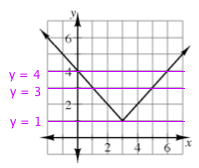### Home > CAAC > Chapter 12 > Lesson 12.1.3 > Problem12-27

12-27.
1. Use the graph of f(x) = | x − 3 | + 1 in problem 12-26 to solve the equations and inequalities below. It may be helpful to copy the graph onto graph paper first. Homework Help ✎

1. | x − 3 | + 1 = 1

2. | x − 3 | + < 4

3. | x − 3 | + 1 = 3

4. | x − 3 | + 1 > 2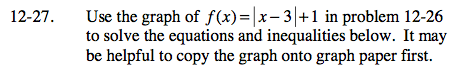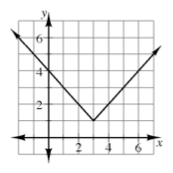Remember that with absolute values, there may be 2 possible solutions.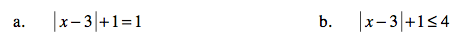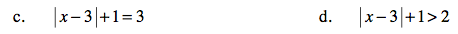Draw line y = 1 onto the graph and find the intersection point(s).

x = 3

See part (a).